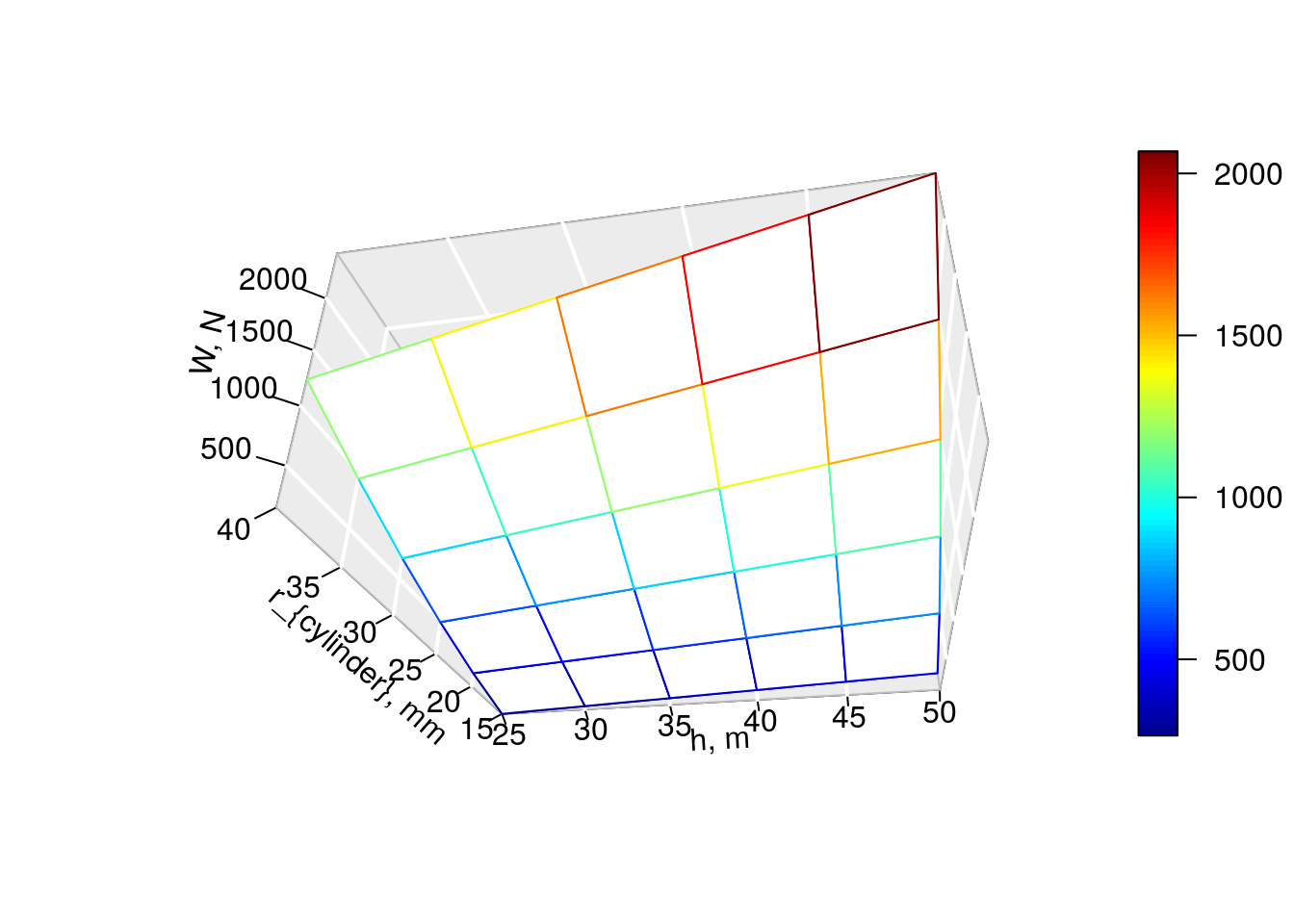Note: If you wish to use any of the libraries noted below, then you will need to copy and paste the following commands in R first:

``````install.packages("install.load") # install the install.load package
# you will need to have GNU Octave (check the RcppOctave CRAN page for more details) installed on your system, then you can use the code below:
install_load("plot3D", "ggplot2", "pracma", "RcppOctave", "reshape2", "directlabels") # install and load the named packages and all of their dependencies, including the extra system dependencies (this process may take a while depending on the number of dependencies)

# Alternatively, if you already have the packages installed, then you can follow the following steps:

The following R code is incomplete as I am still searching for the best way to obtain the same plots in R as with GNU Octave/MATLAB. I posted this question - Q: r - mesh, meshz, surf, and contour plots similar to GNU Octave/MATLAB - online at Stack Overflow, but I have not received an answer yet. Please feel free to post an answer or leave comments. Thank you.

``````# Brockman 470 - 473

# Sources used in the R code
# Source 1
# Statistical tools for high-throughput data analysis (STHDA): Impressive package for 3D and 4D graph - R software and data visualization. See http://www.sthda.com/english/wiki/impressive-package-for-3d-and-4d-graph-r-software-and-data-visualization.

# Source 2
# Fifty ways to draw a volcano using package plot3D by Karline Soetaert. See https://cloud.r-project.org/web/packages/plot3D/vignettes/volcano.pdf.

# Source 3
# ggplot2 - R: How to Label Specific Contours using direct.label - Stack Overflow answered by a different ben on Jul 21 2014. See https://stackoverflow.com/questions/10675387/r-how-to-label-specific-contours-using-direct-label.

# Source 4
# R adding legend and directlabels to ggplot2 contour plot - Stack Overflow answered and edited by Sandy Muspratt on Jul 2 2016. See https://stackoverflow.com/questions/38154679/r-adding-legend-and-directlabels-to-ggplot2-contour-plot.

source("https://raw.githubusercontent.com/ggrothendieck/gsubfn/master/R/list.R")

rho <- 1000

g <- 9.81

r_cylinder <- seq(15, 40, by = 5)

h <- seq(25, 50, by = 5)

# mesh plot

list[r_cylinder_grid, h_grid] <- meshgrid(r_cylinder, h)

W_grid <- rho * g * (pi * (r_cylinder_grid / 1000) ^ 2  * h_grid)

persp3D(r_cylinder_grid, h_grid, W_grid, xlab = "r_{cylinder}, mm", ylab = "h, m", zlab = "W, N", theta = 100, phi = -30, expand = 0.5, ticktype = "detailed", ltheta = 120, bty = "g", facets = FALSE, col = NULL) # Source 1 - 2````````````# meshz plot
# Not available

# surface plot

surf3D(r_cylinder_grid, h_grid, W_grid, xlab = "r_{cylinder}, mm", ylab = "h, m", zlab = "W, N", theta = 100, phi = -30, expand = 0.5, ticktype = "detailed", ltheta = 120, bty = "g", col = gg.col(50)) # Source 1``````